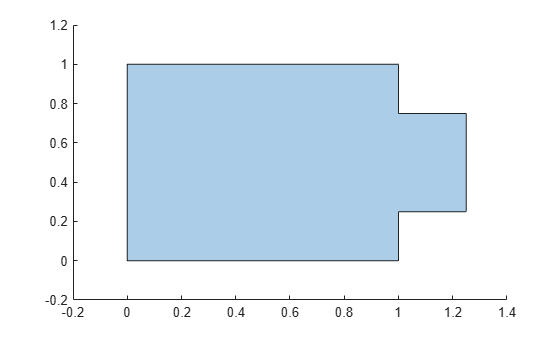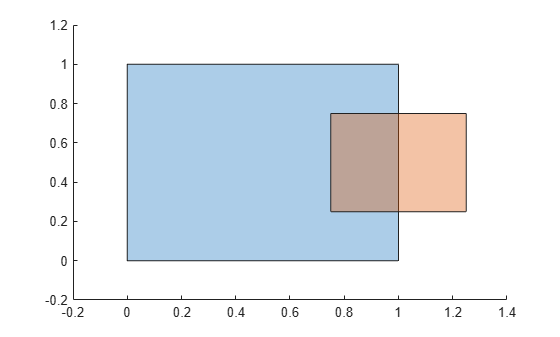union

Union of polyshape objects

Description

example

polyout = union(poly1,poly2) returns a polyshape object whose regions are the union of two polyshape objects. The union contains the combined regions of poly1 and poly2, which must have compatible array sizes.

example

polyout = union(polyvec) returns a polyshape object whose regions are the geometric union of all polyshape objects in the vector polyvec. The union contains the combined regions of the polyshape objects in polyvec.

example

[polyout,shapeID,vertexID] = union(poly1,poly2) also returns vertex mapping information from the vertices in polyout to the vertices in poly1 and poly2. The union function only supports this syntax when poly1 and poly2 are scalar polyshape objects.

The shapeID elements identify whether the corresponding vertex in polyout originated in poly1, poly2, or was created from the union. vertexID maps the vertices of polyout to the vertices of poly1, poly2, or the union.

[polyout,shapeID,vertexID] = union(polyvec) returns vertex mapping information from polyvec to each element of the vector of polyshape objects polyvec.

___ = union(___,'KeepCollinearPoints',TF) specifies whether to keep or remove collinear points in polyout for any of the previous syntaxes.

Examples

collapse all

Create and plot two polygons.

poly1 = polyshape([0 0 1 1],[1 0 0 1]);
poly2 = polyshape([0.75 1.25 1.25 0.75],[0.25 0.25 0.75 0.75]);
plot(poly1)
hold on
plot(poly2)figure

Compute and plot the union of poly1 and poly2.

polyout = union(poly1,poly2)
polyout =
polyshape with properties:

Vertices: [8x2 double]
NumRegions: 1
NumHoles: 0

plot(polyout)
xlim([-0.2 1.4]);
ylim([-0.2 1.2]);Create a vector of polygons and plot each polygon.

polyarray1 = polyshape([0 0 1 1],[1 0 0 1]);
polyarray2 = polyshape([0.75 1.25 1.25 0.75],[0.25 0.25 0.75 0.75]);
poly1 = [polyarray1 polyarray2]
poly1 =
1x2 polyshape array with properties:

Vertices
NumRegions
NumHoles

plot(poly1(1))
hold on
plot(poly1(2))figure

Compute and plot the union of the two polygons.

polyout = union(poly1)
polyout =
polyshape with properties:

Vertices: [8x2 double]
NumRegions: 1
NumHoles: 0

plot(polyout)
xlim([-0.2 1.4]);
ylim([-0.2 1.2]);Create two polygons, and compute and plot their union. Display the vertex coordinates of the union and the corresponding vertex mapping information.

poly1 = polyshape([0 0 1 1],[1 0 0 1]);
poly2 = translate(poly1,[0.5 0]);
[polyout,shapeID,vertexID] = union(poly1,poly2);
plot(polyout)
axis equal[polyout.Vertices shapeID vertexID]
ans = 4×4

0    1.0000    1.0000    1.0000
1.5000    1.0000    2.0000    2.0000
1.5000         0    2.0000    3.0000
0         0    1.0000    4.0000

The first and last vertices of the union originated in poly1, since the corresponding values in shapeID are 1. These vertices are the first and fourth vertices in the property poly1.Vertices, respectively, since the corresponding values in vertexID are 1 and 4. Similarly, the second and third vertices of the union originated in poly2, and they are the second and third vertices in the property poly2.Vertices, respectively.

Input Arguments

collapse all

First input polyshape, specified as a scalar, vector, matrix, or multidimensional array.

Second input polyshape, specified as a scalar, vector, matrix, or multidimensional array.

polyshape vector.

Collinear vertices indicator, specified as false or true:

• false — Remove collinear points so that the output polyshape contains the fewest vertices necessary to define the boundaries.

• true — Keep all collinear points as vertices.

When the 'KeepCollinearPoints' parameter is not specified, its value is assigned according to the values used when creating the input polyshape objects:

• If the value was true for each input polyshape when they were created, then the value for the output polyshape is set to true.

• If the value was false for each input polyshape when they were created, then the value for the output polyshape is set to false.

• If the values for the input polyshape objects do not match, then the value for the output polyshape is set to false.

Data Types: logical

Output Arguments

collapse all

Output polyshape, returned as a scalar, vector, matrix, or multidimensional array.

• If you input two polyshape arguments, then they must have compatible sizes. For example, if two input polyshape vectors have different lengths M and N, then they must have different orientations (one must be a row vector and one must be a column vector). polyout is then M-by-N or N-by-M depending on the orientation of each input vector. For more information on compatible array sizes, see Compatible Array Sizes for Basic Operations.

• If you provide a single input argument polyvec, then polyout is a scalar polyshape object.

Shape ID, returned as a column vector whose elements each represent the origin of the vertex in the union.

• The length of shapeID is equal to the number of rows in the Vertices property of the output polyshape.

• The elements of shapeID depend on the number of input arguments:

• If you provide two input arguments poly1 and poly2, then they must be scalar polyshape objects. The value of an element in shapeID is 0 when the corresponding vertex of the output polyshape was created by the union. An element is 1 when the corresponding vertex originated from poly1, and 2 when it originated from poly2.

• If you provide one input argument polyvec that is a vector of polyshape objects, then shapeID contains the element index of polyvec from which the corresponding output vertex originated. The value of an element is 0 when the corresponding vertex was created by the union.

Data Types: double

Vertex ID, returned as a column vector whose elements map the vertices in the output polyshape to the vertices in the polyshape of origin. The elements of vertexID contain the row numbers of the corresponding vertices in the Vertices property of the input polyshape. An element is 0 when the corresponding vertex of the output polyshape was created by the union.

The length of vertexID is equal to the number of rows in the Vertices property of the output polyshape. If you provide two input polyshape objects, then union only supports this output argument if they are scalar.

Data Types: double